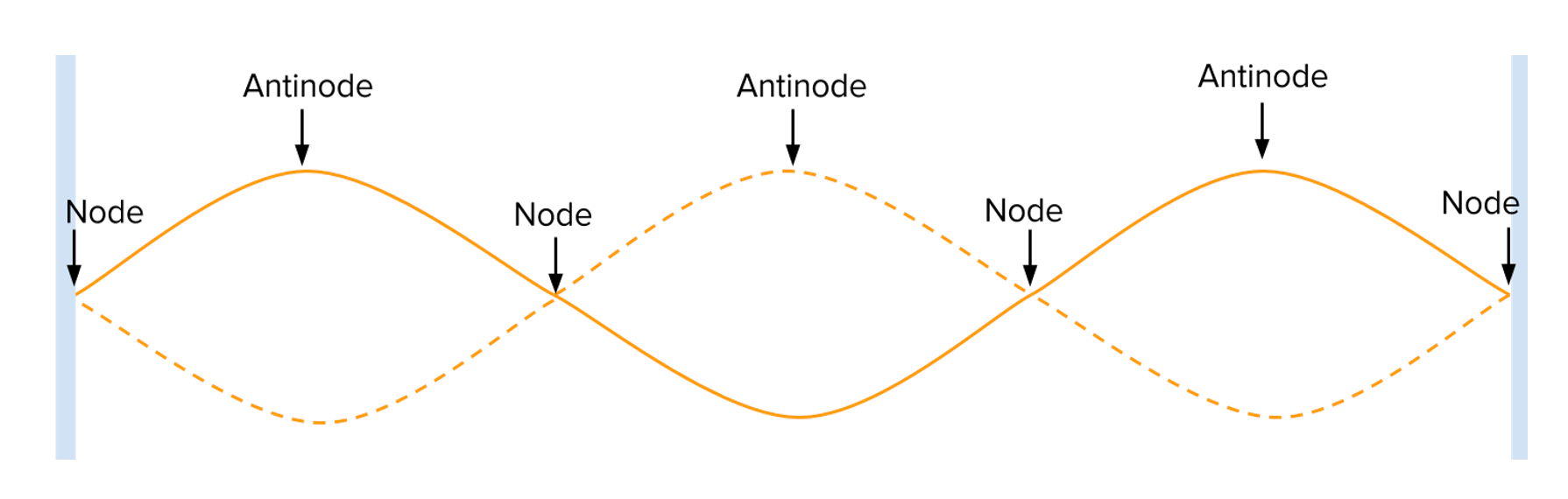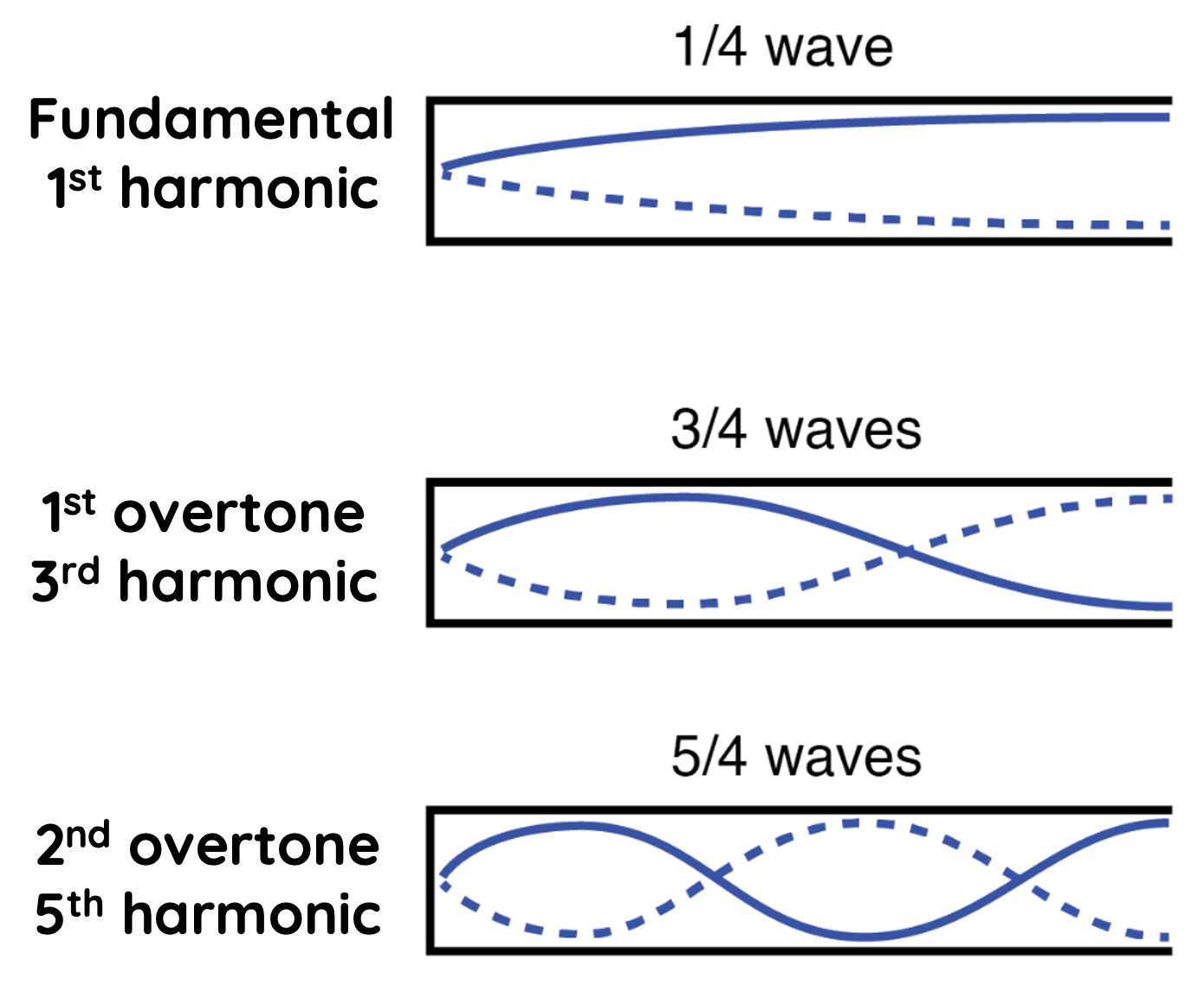# Standing Wave in Pipes

This topic is part of the HSC Physics course under the section Sound Waves.

### HSC Physics Syllabus

• investigate and model the behaviour of standing waves on strings and/or in pipes to relate quantitatively the fundamental and harmonic frequencies of the waves that are produced to the physical characteristics (eg length, mass, tension, wave velocity) of the medium (ACSPH072)

### What are Standing Waves?

Contrary to progressive waves, standing waves, or stationary waves, don't travel or propagate. They form when two waves of the same frequency interfere while moving in opposite directions. The resultant wave appears to "stand still", that is, it continues to oscillate but does not propagate from one place to another.

The following video shows the formation of a standing wave (black) from two progressive waves of the same frequency travelling in the opposite direction. Note that the standing wave oscillates, but does not seem to move to the left nor the right.

Waves do not need to have the same amplitude to produce a standing wave. However, when their amplitudes are equal, the resultant standing wave is the most pronounced, and this is more commonly seen.

Antinodes and nodes of a standing waves are the result of superposition of progressive waves.

• Constructive Interference: At some points, the two waves add up to produce areas of maximum displacement, known as antinodes.

• Destructive Interference: At other points, they cancel each other out, resulting in areas of no displacement, called nodes.This alternating pattern of nodes and antinodes is a hallmark of standing waves.

### How are Standing Waves Formed?

Standing waves are typically formed due to the interference of two waves with the same frequency, travelling in opposite directions within a bounded or confined medium.

This interference can be a result of:

• Reflection: When a wave reflects off a boundary or barrier and interferes with incoming waves of the same kind. For example, a wave moving down a string reflects back upon reaching a fixed end, creating a standing wave.

The production of standing waves using sound in pipes and tubes is discussed separately here.

### Standing Waves on Strings

Standing waves can be produced by the reflection of a progressive wave in a string system that is either fixed or free to move at one end. The frequency of such a progressive wave must be specific to produce the standing wave for reasons we discussed above.

Standing wave on strings is discussed separately here

### Standing Waves in Pipes

Pipe with two open ends

The lowest frequency required to produce a standing wave is the fundamental frequency, f_0, also called the first harmonic.

Frequencies required to form the standing wave are integral multiples of the fundamental frequency:

$$f = nf_0 \, \, \text{where n = 1, 2, 3...}$$The frequency twice the fundamental frequency is called the second harmonic. This is also the first frequency after the fundamental frequency that can produce a standing wave, hence its alternative name, first overtone.

The next frequency (three times the fundamental) is called the third harmonic and second overtone.

The wavelength of the standing wave decreases as its frequency increases such that:

$$\lambda = \frac{2}{n}L \, \, \text{where n = 1, 2, 3...}$$

where L is the length of the pipe.

Standing waves form in a pipe with both ends open such that an antinode (point at which maximum displacement occurs) if present at each open end. The number of nodes (points at which there are no oscillation) also corresponds to the harmonic number (n). For example, a second harmonic standing wave has two nodes.

Pipe with one closed end

Frequencies required to form a standing wave in this system are given by

$$f = nf_0 \, \, \text{where n = 1, 3, 5...}$$

Note that must be an odd integer when one end of the pipe is closed.The first frequency after the fundamental frequency is the third harmonic, which is three times the fundamental frequency.

This also means there are no second (n = 2), fourth (n = 4) harmonics for standing waves in this system.

The wavelength of standing waves is given by

$$\lambda = \frac{4}{n}L \, \, \text{where n = 1, 3, 5...}$$

where L is the length of the pipe.

In a pipe with one open and one closed end, an antinode again occurs at the open end, and a node occurs at the closed end.

### Example

The fundamental frequency of a standing wave in an open pipe is 300 Hz. Calculate:

(a) The length of the pipe

(b) The frequency of the 3rd harmonic

(c) The length required to produce the same fundamental frequency if the pipe is closed.

Solution to part (a):

The wavelength is given by

$$\lambda = \frac{v}{f}$$

$$\lambda = \frac{340}{300} = 1.13 \, \text{m}$$

The relationship between wavelength and the length of the open pipe for the first harmonic is given by

$$\lambda = 2L$$

$$L = 0.57 \, \text{m}$$

Solution to part (b):

In an open pipe, the frequency of the third harmonic is three times the fundamental frequency:

$$f = 3 \times 300 = 900 \, \text{Hz}$$

Solution to part (c):

The relationship between wavelength and length of a closed pipe for the fundamental frequency is given by

$$lambda = 4L$$

$$L = 0.283 \, \text{m}$$